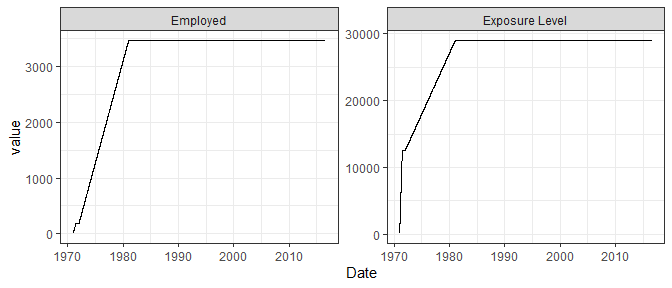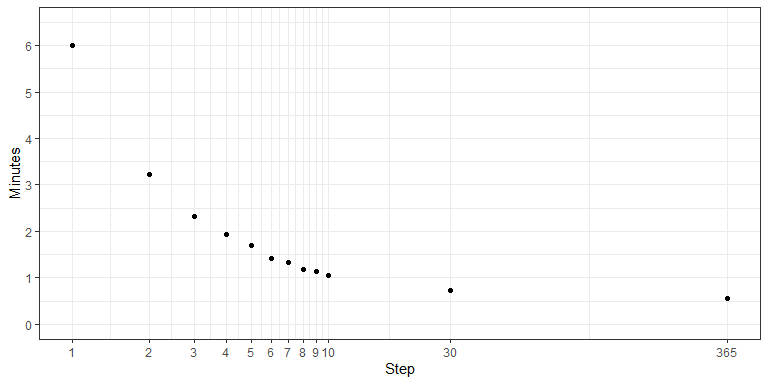# History File Stratification

## History File

In order to stratify a cohort by a time-dependent exposure covariate (aside from age and calendar period), a history file must be created and read in. This file contains one row per person per exposure period. An exposure period is a period of time in which all daily values/levels of an exposure variable are assumed to be constant.

Below are the required variables to be found within the history file:

Variable Description Format
id Unique identifier for each person
begin_dt Beginning date of exposure period character
end_dt End date of exposure period character
<daily exposure variables> Exposure variable(s) numeric

Below is an example layout of a history file with multiple exposures:

id begin_dt end_dt employed exposure_level
1 12/21/1970 6/15/1971 1 71.0
1 6/16/1971 12/31/1980 1 5.0
2 1/19/1972 6/15/1975 1 10.0
3 11/23/1970 12/7/1972 1 41.5

The above example contains 3 persons with 2 exposure variables, employed and exposure_level. Person/id 1 contains 2 non-overlapping exposure periods in which employed is 1 for both but exposure_level drops from 71 to 5 units per day.

## Calculation of Cumulative Exposure From History File

LTASR comes with an example history file, called history_example, that can be used in conjunction with person_example for testing. Below reads in both example files and formats dates appropriately:

person <- person_example %>%
mutate(dob = as.Date(dob, format='%m/%d/%Y'),
pybegin = as.Date(pybegin, format='%m/%d/%Y'),
dlo = as.Date(dlo, format='%m/%d/%Y'))

history <- history_example %>%
mutate(begin_dt = as.Date(begin_dt, format='%m/%d/%Y'),
end_dt = as.Date(end_dt, format='%m/%d/%Y')) %>%
group_by(id)

For the remainder of this section, we will consider Person/id 1 to demonstrate how exposure is calculated over time. Below is the information found within the person file for person/id 1:

id gender race dob pybegin dlo vs rev code
1 M W 11/20/1945 12/21/1970 7/31/2016

This example person’s follow-up starts on 12/21/1970 and continues through 7/31/2016. Below plots their cumulative exposure for both employed and exposure_level variables:Both exposures start at 0, then employed increases by 1 unit per day for both periods. This can therefore be thought of as a duration variable (in days) of all periods. The exposure_level increases rapidly (71 units per day) during the first period and then increases slower (5 units per day).

NOTE: Any gaps within the history file and the follow-up times (for example, the period between the last exposure period within the history file and through the end of follow-up) is assumed to be 0. That is, exposure values do not change during these periods.

## Stratifying person time

Once the person file and history file have been read in (see Demo for basic stratification vignette for additional information on how to read in files), information on how to stratify the exposure variables must be defined using the exp_strata() function.

Below specifies which exposure variables to consider, what cut-points to use for stratification and any lag (in years) to apply to the cumulative exposure variable:

exp1 <- exp_strata(var = 'employed',
cutpt = c(-Inf, 365, Inf),
lag = 0)
exp2 <- exp_strata(var = 'exposure_level',
cutpt = c(-Inf, 0, 10000, 20000, Inf),
lag = 10)

The employed variable will contain 2 strata: (-Inf, 365] and (365, Inf]. Or, put alternatively, ≤ 1 year and > 1 year.

The exposure_level will contain 5 strata: (-Inf, 0], (0, 10000], (10000, 20000] and (20000, Inf). Therefore, the first category defines unexposed person-time. Additionally, a 10 year lag will be applied when defining strata.

Once the exposure strata have been defined, LTASR provides two functions for stratifying the cohort. One is get_table_history whose usage is:

py_table <- get_table_history(persondf = person,
rateobj = us_119ucod_19602020,
historydf = history,
exps = list(exp1, exp2))

This creates the below table:

ageCat CPCat gender race employedCat exposure_levelCat pdays _o55 _o52
[15,20) [1970,1975) F W (-Inf,365] (-Inf,0] 365 0 0
[15,20) [1970,1975) F W (365, Inf] (-Inf,0] 381 1 0
[25,30) [1970,1975) M N (-Inf,365] (-Inf,0] 55 0 0
[25,30) [1970,1975) M W (-Inf,365] (-Inf,0] 177 0 0
[25,30) [1970,1975) M W (365, Inf] (-Inf,0] 1295 0 0
[25,30) [1975,1980) M W (365, Inf] (-Inf,0] 323 0 0

This function is very fast, and replicates how the original LTAS behaved. It also exactly stratifies the person-days into the appropriate strata. However, it may be desired to calculate mean exposure values for each strata to be used in a Poisson regression later. To implement this exactly is very slow.

Therefore, a separate function, get_table_history_est, calculates these mean exposure values and also allows for a step parameter to be specified defining the number of days to calculate the cumulative exposure.

An example usage is:

py_table_est <- get_table_history_est(persondf = person,
rateobj = us_119ucod_19602020,
historydf = history,
exps = list(exp1, exp2),
step = 7)

By specifying step = 7, person time is considered every 7 days when allocating person-time to strata. This results in a significant increase in speed at the cost of a (generally) trivial amount of inaccuracy.

Specifying step = 1 will calculate strata exactly for each individual day, but is significantly slower.

Below is the result of this specification:

ageCat CPCat gender race employedCat exposure_levelCat pdays _o55 _o52 employed exposure_level
[15,20) [1970,1975) F W (-Inf,365] (-Inf,0] 361 0 0 184.0 0
[15,20) [1970,1975) F W (365, Inf] (-Inf,0] 385 1 0 557.0 0
[25,30) [1970,1975) M N (-Inf,365] (-Inf,0] 53 0 0 30.0 0
[25,30) [1970,1975) M W (-Inf,365] (-Inf,0] 376 0 0 136.9 0
[25,30) [1970,1975) M W (365, Inf] (-Inf,0] 1096 0 0 927.5 0
[25,30) [1975,1980) M W (365, Inf] (-Inf,0] 322 0 0 1636.5 0

As can be seen, the pday are slightly different than the previous table. However, the effects on results will generally be trivial.

In addition, two additional variables are available: employed and exposure_level indicating the person-time weighted mean values.
## Step Specifications

When specifying the step parameter, there is a trade-off between computation speed and accuracy. Specifying step = 1 will result in the most accurate stratification, but can be extremely slow.

To investigate this further, below plots the time (in minutes) taken to stratify a cohort of 5,200 people with 2 exposure variables for various specifications of the step parameter:There are dramatic savings in computation time when increasing the step parameter in the low end. In this example, at about step = 10, improvements in computation time diminishes. It seems a step parameter of about 5-10 is a good compromise.

Exact times will depend upon:

• the size of the cohort,
• the number of exposure variables and
• the the number of strata per exposure variable.

An additional consideration is the level of detail of the exposure variable. That is, if exposure is dramatically changing, relative to its specified strata, the loss of accuracy will be more dramatic for small increases of the step parameter. For example, age is stratified by 5-year increments, therefore, a step value of 1-week (step = 7) will cause a trivial amount of inaccuracy.

One option is to use a crude step value during initial investigations, but when results are to be published/presented, the function can be run again with a smaller step value.